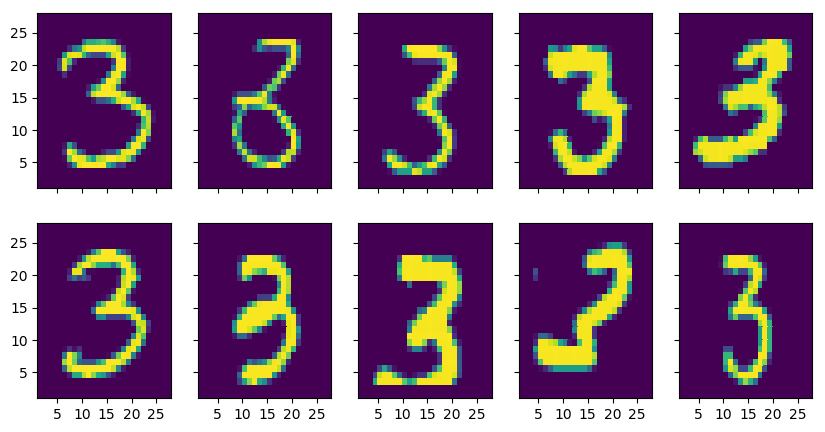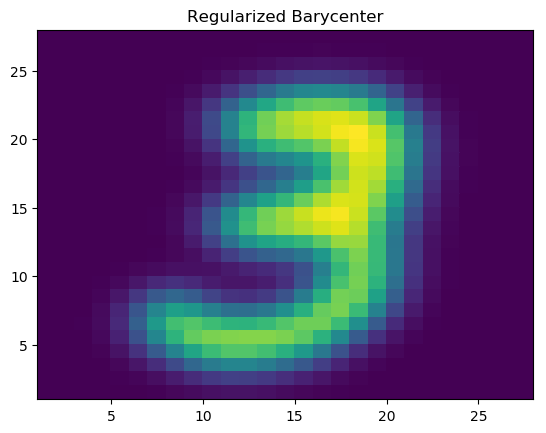Implementing Regularised Wasserstein Barycenter problem using JuMP in Julia.¶

The goal of this notebook is to implement a model to calculate the Wasserstein barycenter by solving an entropy regularised minimization problem in Julia with JuMP and then solve it using MosekTools. For additional info about the data used, theoretical explanation of the calculation of barycenters, references and for more insight in construction of the model, please consult the corresponding Python notebook. Data files can be found in http://yann.lecun.com/exdb/mnist/.

In :
using LinearAlgebra
using Plots
pyplot()

using JuMP

using Mosek
using MosekTools

using Statistics
In :
#Define the number of images for the barycenter calculation.
n = 2

#Read the images from the file.
f = open(filename,"r")
data_layout = zeros(UInt8,4)
data_zero = reinterpret(UInt16,data_layout[1:2])
data_type,data_dimensions = reinterpret(UInt8,data_layout[3:4])
data_shape = Int32[]
for i = 1:data_layout
s = zeros(UInt8,4)
s = map(hton,reinterpret(Int32,s))
push!(data_shape,s)
end
idx_data = zeros(UInt8,cumprod(data_shape)[length(data_shape)])
idx_data = reshape(idx_data,Tuple(reverse(data_shape)))
return(idx_data)
end

#Select the images.
train = train_ones[:,:,1:n]

x = [i for i=1:28]
y = reverse(x)
f,ax = PyPlot.plt.subplots(2,5,sharey=true,sharex=true,figsize=(10,5))
PyPlot.plt.xticks([5,10,15,20,25])

for i = 1:10
rand_pick = rand(1:size(train_ones))
ax[i].pcolormesh(x,y,transpose(train_ones[:,:,rand_pick]))
endIn :
function single_pmf(data)
#Takes a list of images and extracts the probability mass function.
v = vec(data[:,:,1])
v = v./cumsum(v)[length(v)]
for im_k in 2:size(data)
image = data[:,:,im_k]
arr = vec(image)
v_size = size(arr)
v = hcat(v, arr./cumsum(arr)[length(arr)])
end
return v,size(v)
end

function ms_distance(m,n)
#Squared Euclidean distance calculation between the pixels.
d = ones(m,m)
coor_I = []
for c_i in 1:n
append!(coor_I,ones(Int,n).*c_i)
end
coor_J = repeat(1:n,n)
coor = hcat(coor_I,coor_J)
for i in 1:m
for j in 1:m
d[i,j] = norm(coor[i,:]-coor[j,:]).^2
end
end
return d
end

function reg_wasserstein_barycenter(data,lambda,relgap)
#Calculation of wasserstein barycenter by solving an entropy regularised minimization problem.
#Direct mode model
#M = direct_model(Mosek.Optimizer(MSK_DPAR_INTPNT_CO_TOL_REL_GAP=relgap))

#Automatic mode model
M = Model(with_optimizer(Mosek.Optimizer,MSK_DPAR_INTPNT_CO_TOL_REL_GAP=relgap))

if length(size(data))==3
K = size(data)
else
K = 1
end
v,N = single_pmf(data)
d = ms_distance(N,size(data))

if lambda==nothing
lambda = 60/median(vec(d))
end

#Define indices
M_i = 1:N
M_j = 1:N
M_k = 1:K

M_pi = @variable(M, M_pi[i = M_i, j = M_j, k = M_k] >= 0.0)
M_mu = @variable(M, M_mu[i = M_i] >= 0.0)

#Auxiliary variable for the conic constraint
M_aux = @variable(M,M_aux[i = M_i, j = M_j, k = M_k])

@constraint(M, c3_expr[k = M_k, j = M_j], sum(M_pi[:,j,k]) == v[j,k])
@constraint(M, c2_expr[k = M_k, i = M_i], sum(M_pi[i,:,k]) == M_mu[i])

@constraint(M,cExp_cone[i=M_i, j=M_j, k=M_k],[M_aux[i,j,k],M_pi[i,j,k],1] in MOI.ExponentialCone())

#Non-linear objective in the case of Regularized barycenters.
W_obj = @objective(M, Min,(sum(d[i,j]*M_pi[i,j,k] for i=M_i,j=M_j,k=M_k) -
sum(M_aux[i,j,k] for i=M_i,j=M_j,k=M_k)/lambda)/K)

return M,M_mu
end
Out:
reg_wasserstein_barycenter (generic function with 1 method)
In :
function run_regularised_model(data,lambda=nothing,relgap=1e-7)
@time begin
M,M_mu = reg_wasserstein_barycenter(data,lambda,relgap)
optimize!(M)
end
println("Solution status = ",termination_status(M))
println("Primal objective value = ",objective_value(M))
mu_level = value.(M_mu)
return mu_level
end

function show_barycenter(bary_center)
bary_center = reshape(bary_center,(28,28))
x = [i for i=1:28]
y = reverse(x)
PyPlot.plt.pcolormesh(x,y,transpose(bary_center))
PyPlot.plt.title("Regularized Barycenter")
PyPlot.plt.show()
end
Out:
show_barycenter (generic function with 1 method)
In :
bary_center = run_regularised_model(train)
println("******")
Problem
Name                   :
Objective sense        : min
Type                   : CONIC (conic optimization problem)
Constraints            : 3691072
Cones                  : 1229312
Scalar variables       : 6147344
Matrix variables       : 0
Integer variables      : 0

Optimizer started.
Presolve started.
Linear dependency checker started.
Linear dependency checker terminated.
Eliminator started.
Freed constraints in eliminator : 0
Eliminator terminated.
Eliminator - tries                  : 1                 time                   : 0.00
Lin. dep.  - tries                  : 1                 time                   : 1.02
Lin. dep.  - number                 : 1
Presolve terminated. Time: 7.65
Problem
Name                   :
Objective sense        : min
Type                   : CONIC (conic optimization problem)
Constraints            : 3691072
Cones                  : 1229312
Scalar variables       : 6147344
Matrix variables       : 0
Integer variables      : 0

Optimizer  - solved problem         : the primal
Optimizer  - Constraints            : 1138
Optimizer  - Cones                  : 1229312
Optimizer  - Scalar variables       : 3687936           conic                  : 3687936
Optimizer  - Semi-definite variables: 0                 scalarized             : 0
Factor     - setup time             : 2.62              dense det. time        : 0.00
Factor     - ML order time          : 0.01              GP order time          : 0.00
Factor     - nonzeros before factor : 2.79e+05          after factor           : 3.41e+05
Factor     - dense dim.             : 0                 flops                  : 1.17e+08
ITE PFEAS    DFEAS    GFEAS    PRSTATUS   POBJ              DOBJ              MU       TIME
0   6.3e+02  5.1e+02  2.4e+07  0.00e+00   2.213290906e+07   -1.586952925e+06  1.0e+00  16.82
1   2.2e+02  1.8e+02  1.4e+07  -9.87e-01  1.997448319e+07   -3.251325127e+06  3.5e-01  21.07
2   1.4e+02  1.1e+02  1.0e+07  -8.50e-01  1.706335688e+07   -4.132584961e+06  2.2e-01  26.16
3   8.6e+01  6.9e+01  6.1e+06  -5.35e-01  1.255536087e+07   -4.350508428e+06  1.4e-01  33.89
4   3.9e+01  3.1e+01  2.3e+06  -5.91e-02  6.634369363e+06   -3.290261645e+06  6.2e-02  38.48
5   1.6e+01  1.3e+01  6.8e+05  4.56e-01   3.047700570e+06   -1.738022157e+06  2.5e-02  42.26
6   9.2e+00  7.4e+00  3.2e+05  6.93e-01   1.871313131e+06   -1.107859520e+06  1.5e-02  45.87
7   3.8e+00  3.0e+00  9.0e+04  7.82e-01   8.078383522e+05   -5.102774510e+05  5.9e-03  50.28
8   1.0e+00  8.1e-01  1.4e+04  8.51e-01   2.027574293e+05   -1.783130565e+05  1.6e-03  54.31
9   4.2e-01  3.4e-01  4.0e+03  8.87e-01   8.245554128e+04   -8.500164850e+04  6.6e-04  58.08
10  1.2e-01  9.9e-02  6.9e+02  9.00e-01   2.254804899e+04   -2.962342498e+04  2.0e-04  61.72
11  3.6e-02  2.9e-02  1.2e+02  9.02e-01   5.918580033e+03   -1.024328330e+04  5.7e-05  65.07
12  1.1e-02  8.5e-03  2.0e+01  9.00e-01   1.600621502e+03   -3.421588743e+03  1.7e-05  68.39
13  3.3e-03  2.6e-03  3.7e+00  9.03e-01   4.338656334e+02   -1.203318166e+03  5.2e-06  71.91
14  9.2e-04  7.4e-04  5.9e-01  9.15e-01   9.099381179e+01   -3.977761008e+02  1.5e-06  75.28
15  2.5e-04  2.0e-04  8.5e-02  9.22e-01   1.264148878e+00   -1.351942993e+02  3.9e-07  78.65
16  7.1e-05  5.7e-05  1.4e-02  9.31e-01   -1.815177735e+01  -5.892407032e+01  1.1e-07  81.93
17  1.6e-05  1.3e-05  1.6e-03  9.35e-01   -2.349891579e+01  -3.326506346e+01  2.6e-08  85.27
18  3.2e-06  2.6e-06  1.5e-04  9.38e-01   -2.441821029e+01  -2.642392235e+01  5.1e-09  88.70
19  7.5e-07  6.0e-07  1.7e-05  9.44e-01   -2.452958618e+01  -2.501499156e+01  1.2e-09  92.04
20  1.8e-07  1.4e-07  2.0e-06  9.49e-01   -2.454255521e+01  -2.466071465e+01  2.8e-10  95.32
21  3.6e-08  2.9e-08  1.9e-07  9.48e-01   -2.454079676e+01  -2.456580776e+01  5.7e-11  99.05
22  9.3e-09  7.5e-09  2.6e-08  9.50e-01   -2.453852209e+01  -2.454516580e+01  1.5e-11  102.62
23  2.3e-09  1.8e-09  3.3e-09  9.51e-01   -2.453774799e+01  -2.453943385e+01  3.6e-12  106.06
24  8.2e-10  5.0e-10  4.7e-10  9.51e-01   -2.453754484e+01  -2.453801397e+01  9.8e-13  109.44
25  8.0e-10  4.1e-10  3.6e-10  9.54e-01   -2.453752652e+01  -2.453792006e+01  8.1e-13  120.02
26  1.3e-09  3.7e-10  3.1e-10  9.54e-01   -2.453751783e+01  -2.453787528e+01  7.4e-13  135.61
27  1.2e-09  3.6e-10  2.9e-10  9.54e-01   -2.453751378e+01  -2.453785435e+01  7.0e-13  149.61
28  1.4e-09  3.4e-10  2.7e-10  9.54e-01   -2.453750992e+01  -2.453783439e+01  6.7e-13  161.23
29  1.4e-09  3.1e-10  2.3e-10  9.54e-01   -2.453750254e+01  -2.453779623e+01  6.0e-13  173.52
30  1.4e-09  2.9e-10  2.1e-10  9.54e-01   -2.453749919e+01  -2.453777893e+01  5.7e-13  186.35
31  1.4e-09  2.8e-10  2.1e-10  9.54e-01   -2.453749759e+01  -2.453777069e+01  5.6e-13  199.37
32  1.4e-09  2.8e-10  2.1e-10  9.54e-01   -2.453749749e+01  -2.453777019e+01  5.6e-13  215.66
33  1.4e-09  2.8e-10  2.1e-10  9.54e-01   -2.453749746e+01  -2.453777006e+01  5.6e-13  233.18
34  1.4e-09  2.8e-10  2.0e-10  9.54e-01   -2.453749669e+01  -2.453776604e+01  5.5e-13  246.21
35  1.4e-09  2.8e-10  2.0e-10  9.54e-01   -2.453749649e+01  -2.453776505e+01  5.5e-13  261.34
36  1.5e-09  2.8e-10  2.0e-10  9.54e-01   -2.453749644e+01  -2.453776480e+01  5.5e-13  279.47
37  1.5e-09  2.8e-10  2.0e-10  9.54e-01   -2.453749643e+01  -2.453776474e+01  5.5e-13  298.25
38  1.5e-09  2.8e-10  2.0e-10  9.54e-01   -2.453749641e+01  -2.453776462e+01  5.5e-13  315.76
39  1.5e-09  2.8e-10  2.0e-10  9.54e-01   -2.453749640e+01  -2.453776460e+01  5.5e-13  335.01
40  1.5e-09  2.8e-10  2.0e-10  9.54e-01   -2.453749640e+01  -2.453776457e+01  5.5e-13  354.66
41  1.5e-09  2.8e-10  2.0e-10  9.54e-01   -2.453749640e+01  -2.453776457e+01  5.5e-13  373.38
42  1.5e-09  2.8e-10  2.0e-10  9.54e-01   -2.453749640e+01  -2.453776457e+01  5.5e-13  392.86
Optimizer terminated. Time: 419.08

649.126111 seconds (508.56 M allocations: 33.802 GiB, 22.71% gc time)
Solution status = SLOW_PROGRESS
Primal objective value = -24.5374963989306
******
In :
show_barycenter(bary_center)